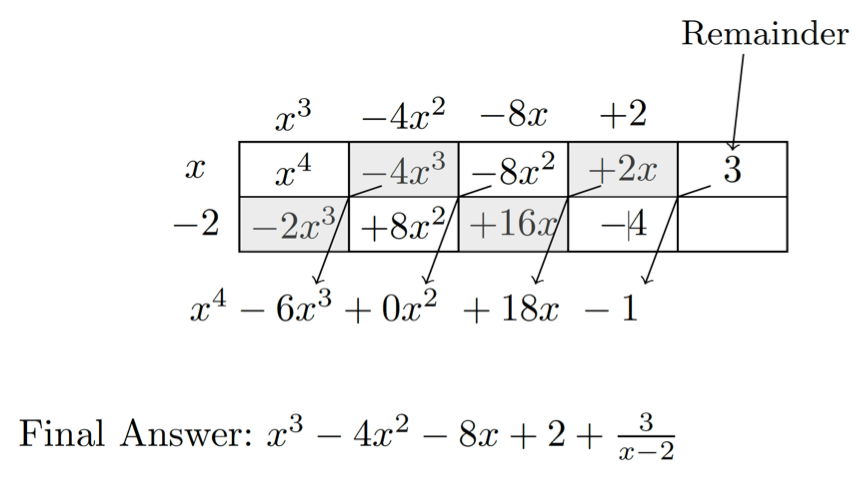# POLQ 1 | Lesson 2 | Explore (Dividing using Generic Rectangles)# Dividing using Generic Rectangles

There is a third way to divide polynomial using generic rectangles like we did with multiplication.

Example:

$$\dfrac{x^4-6x^3+18x-1}{x-2}$$

To use generic rectangles for division of polynomials, follow these steps:

1. Place the terms of the divisor on the outside or the rectangle.
2. Place the first term of the dividend in the top left hand box. Divide the first term of the divisor into the first term of the dividend.
3. Place the quotient on top of the first column. Multiply it by the remainder of the terms in the divisor and place those products in the first column.
4. Each of the products is subtracted from the next term in the dividend. In other words, the diagonals should add to the successive terms in the dividend.
5. Any number left in the final column is a remainder.Examples of polynomial division with a divisor of three terms can be found on the link below.

Dividing Polynomials – The Grid Method | Mathrecreation